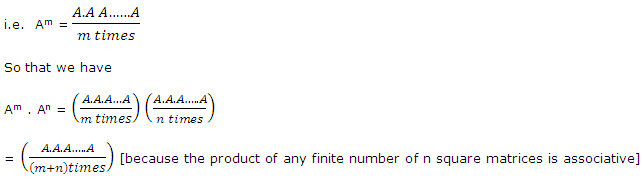# Describe the positive integral powers of matrices

Publish On: 2019-03-09

Total Post: 542

# Mjay Jollay

Total Post: 0

## ANS: Describe the positive integral powers of matrices

We have seen that the product A.A is defined if and only if A is a square matrix. Let A be n square matrix. We denote the product A.A by A2. Obviously A2 is an n × n matrix. Again the product A2.A is defined and is an n × n matrix.

Also A2.A (A.A)A = A.(A.A) = A.A2       [By Associative law]

so that A2.A = A.A2 = A.A.A = A3.

We write A3 = A2.A = A.A2 = A.A.A.

By the generalized associative law, the product of any finite numbers of matrices is associative and hence we can write down the product A.A.A, ….., A m times equal to Am.= Am+n

Similarly, we have

(Am)n = Amn.